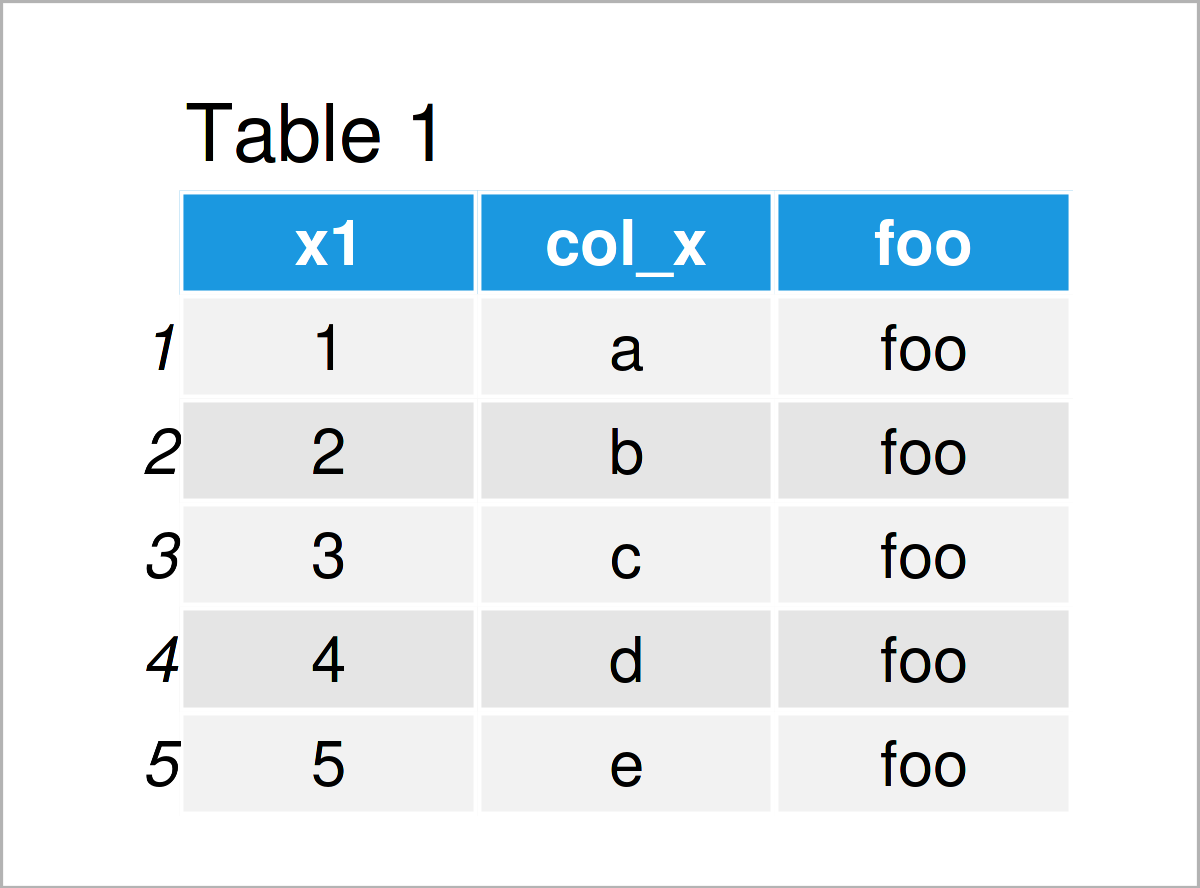# Get Column Names of Data Frame in R (2 Examples)

This post demonstrates how to extract the column names from a data frame in R.

Let’s do this.

## Creating Example Data

At first, let’s define some example data frame:

```data <- data.frame(x1 = 1:5,      # Create example data frame
col_x = letters[1:5],
foo = "foo")
data                              # Print example data frame```Have a look at the table that got returned after executing the previous code. It visualizes that our example data is made of five lines and the three variables “x1”, “col_x”, and “foo”.

Let’s extract the column names from this example data frame using some R functions!

## Example 1: Extract Column Names from Data Frame Using colnames() Function

Example 1 shows how to return the variable names from a data frame using the colnames function.

Have a look at the following R syntax:

```my_colnames1 <- colnames(data)    # Apply colnames function
my_colnames1                      # Print vector of column names
#  "x1"    "col_x" "foo"```

The previous RStudio console output shows that we have created a new vector object that contains the three column names of our data frame as character strings.

Note that the colnames function could also be used to extract the column names of a matrix object.

## Example 2: Extract Column Names from Data Frame Using names() Function

Alternatively to the colnames function, we may also use the names function to get the column names of a data frame:

```my_colnames2 <- names(data)       # Apply names function
my_colnames2                      # Print vector of column names
#  "x1"    "col_x" "foo"```

As you can see, the output is exactly the same as in Example 1.

## Video & Further Resources

Have a look at the following video on my YouTube channel. I show the content of this article in the video.

Please accept YouTube cookies to play this video. By accepting you will be accessing content from YouTube, a service provided by an external third party.If you accept this notice, your choice will be saved and the page will refresh.

Besides the video, you may read the other articles on this website. A selection of tutorials that are related to the extraction of the variable names of a data frame can be found below:

In this R tutorial you have learned how to get the variable names of a data frame. Let me know in the comments below, if you have any additional comments or questions.

Subscribe to the Statistics Globe Newsletter